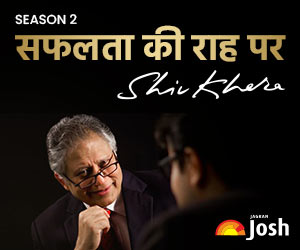# NCERT Solutions for Class 10 Maths Chapter 9 - Some Applications of Trigonometry (PDF)

Here, we are providing accurate solutions to all questions of Class 10 Maths Chapter 9 - Some Applications of Trigonometry. These solutions are best to clear concepts and prepare well for the exams.Class 10 Maths NCERT Solutions

In this article, you will get the NCERT solutions for all the questions given in CBSE Class 10 Mathematics Chapter 9 - Some Applications of Trigonometry. These model solutions provide in-depth and step-by-step solutions to all questions given in the NCERT textbooks and are an irreplaceable support to students that will help them in making an effective preparation for the board exams 2021-2022.

All the solutions are explained by subject experts. Students having trouble solving tough Math problems can refer to these NCERT solutions.

Main topics discussed in Class 10 Mathematics chapter- Some Applications of Trigonometry are:

• Main concepts used in applications of trigonometry
• Heights and Distances and

Students may download all the NCERT Solutions for CBSE Class 10 Mathematics chapter – Some Applications of Trigonometry, in the form of PDF.Some of the questions and their solutions from NCERT Solutions for Class 10: Some Applications of Trigonometry are as follows:

Get the complete the NCERT Chapter: Some Applications of Trigonometry and its NCERT solution from the following links:

 Some Applications of Trigonometry NCERT Book Chapter NCERT Solutions

If you want to get the detailed, precise and free solutions for CBSE Class 10 Mathematics NCERT Book, then jagranjosh.com, India’s number one education website brings here the accurate solutions to all the questions given in the NCERT textbook.

Our experts in Maths have reviewed the detailed NCERT solutions for class 10 Mathematics. Solving the class 10 Maths book questions becomes easy when done with the help of the solutions provided here.

Key points of NCERT Solutions:

• The NCERT Solutions provided here are absolutely free
• Thorough and modified answers are provided for each question given in the NCERT textbook
• Created by subject matter experts